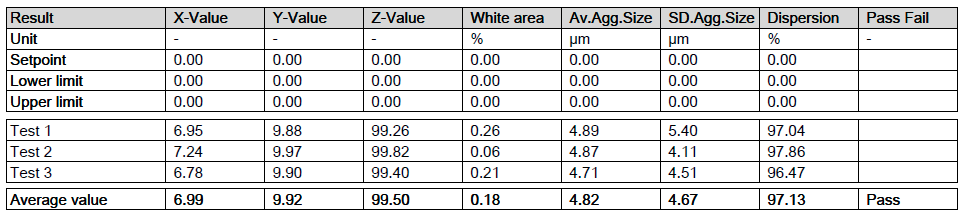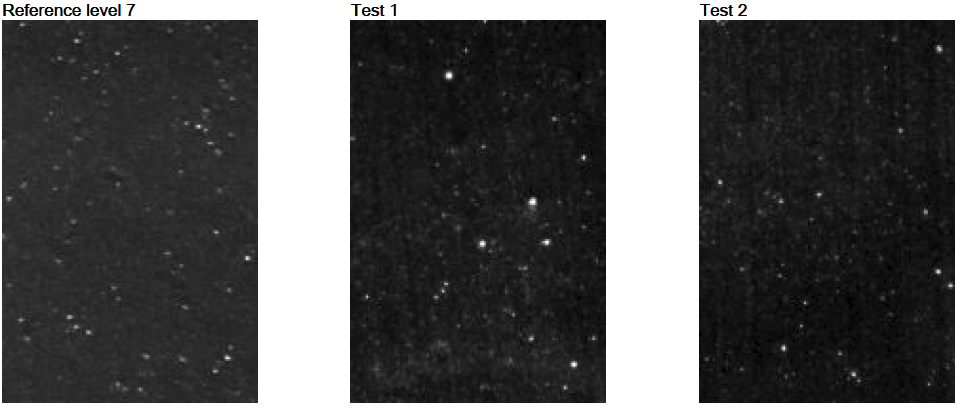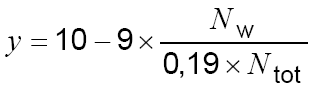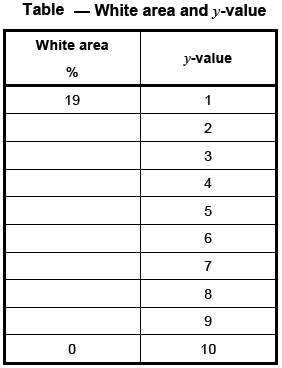Optical Rubber Dispersion Testing Data Points Explained:

Optical dispersion testing is one of the fastest ways to view a basic blueprint of mix quality. This is quantified by placing a sample in front of the aperture of the DisperTester+ where then the unit will ascertain the count and size of the filler agglomerates found through the compound. These agglomerates, depending on the size and frequency, can directly affect physical properties and processability of the compound.

This MonTech Tips guide explains the primary data points found within a DisperTester+ Report

The DisperTester+ reports the following data points with every finished test:Three tests were completed on a carbon black filled NBR, yielding average values in seven categories seen above. Let's unpack each data point:

1. What is the X-Value?

The X-Value is a comparison of the size and frequency of any agglomerates in the test piece (showing up as surface bumps or depressions) with those in reference photographs also found in every DisperTester+ report. The X-Value is a grade from 1 to 10. 10 being perfectly dispersed and 1 being poorly dispersed.2. What is the Y-Value?

Y-Value is also a numerical value from 1-10 (see table below) where 10 represents the total absence of agglomerates with a diameter of 23μm or larger, and 1 represents a large number of such agglomerates (corresponding to 19% of the test area), and is calculated from the equation:

Nw: The number of white pixels in the image of the test area due to nodules with an average diameter of 23μm. or larger caused by underlying agglomerates.

Ntot: The total number of pixels in the image of the test area.3. What is the Z-Value?

The Z-Value is a simple value for quantifying dispersion, ranking from 100 (perfect mix) to 0 (poorer mix) and being proportional to the amount of  undispersed agglomerates.

This method is independent of any reference scale.

4. What is White Area %?

100 / [Sum of all image pixels] X [Sum of all pixels in a agglomerated white area >= 23 μm]

5. What is Av. Agg. Size?

Average particle diameter in μm.

6. What is the SD. Agg. Size?

Standard of deviation to Av. Agg. Size

7. What is Dispersion %?

100 - (100 /  [Sum of all image pixels] x [Sum of all agglomerate pixels])

The Dispersion % Calculation includes the whole agglomerate size range from 2 to 62 μm.